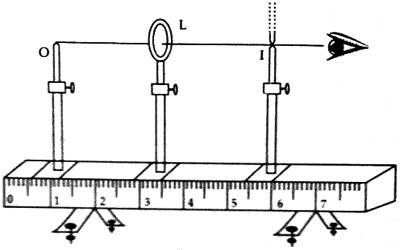# Experiment: Determination of the Focal Length and Power of a Lens

Experiment: Determination of the Focal Length and Power of a Lens

The power of a lens is determined by its ability to create a difference of light. A lens of short focal length produces more deviation of light than that of a lens of larger focal length. So, the power of a lens is defined as the reciprocal of its focal length.

To determine the focal length and power of a convex lens by plotting 1/u and 1//v graph.

Theory: Focal length: The distance between the optical centers to the principal focus of a lens is called focal length of that lens and it is expressed by ƒ. If the focal length of a lens is expressed in meter and sign of its inverse value is changed then the power of the lens is obtained in dioptre. If u and v are respectively the object distance and image distance respectively then for real image we get –

1/ƒ = 1/v + 1/u

Now if a curve is drawn by 1/u in the Y-axis and 1//v in the X-axis then the curve will be a straight line. The straight line will intersect the axes at equal distance from the origin. The distance of the point of intersection on both the axes from the origin is equal to 1/ƒ.

As in figure, at X-axis, 1/v = 0, so the equations, 1/v + 1/u = 1/ƒ, can be written as 1/u + 0= 1/ƒ; or, u = ƒ. Similarly on Y-axis, 1/u = 0, so, 1/v + 1/u = 1/ƒ, equation becomes, 1/v + 1/0 = 1/ƒ, or, v = ƒ. But if the distance of the intersections are not equal, then avenge value is to be taken.

After determining the focal length, the power of the lens can be determined by the following equation.

Power of a lens: The power of divergence or convergence of light rays by a lens is called its power. As the focal length of the lens is less, the less is the distance within which parallel rays become converge or diverge. That means a power of that lens is more.

That is why the inverse of the focal length of a lens is called its power. So, if the focal length of a lens is known the power of that lens can be determined by the following equation.

Power of a lens, P = 100/ƒ(cm) Diopter (D) or 1/ƒ

or, P = 1/ƒ(cm) Diopter (D).

Since the lens is a convex lens, so its power will be positive.

Apparatus and other necessary materials: Optical bench, Experimental convex leas, Object pin, Screen pin, Index rod, Graph paper etc.Procedure:

1. A convex lens is taken and it is plated on a rod placed on the optical bench,
2. In front of the lens and on the optical bench a pin is placed on another rod.
3. On the other side of the lens, a pin placed on a rod is placed on the optical bench in such a way as the real image of the first pin becomes incident on it avoiding the parallax error.
4. From the scale of the optical bench positions at the lens, object-pin and image pin are taken and apparent values of u and t, are found out.
5. A few more apparent values of u and v found out following the above procedures.
6. The index error of the object distance and image distance are found out and apparent values of u and v are found out.
7. In each case values of 1/u and 1/v are determined.

A graph is drawn taking 1/u along X-axis and 1/v along Y-axis. The distance from the origin to the points that the straight line intercepts on X and Y-axis is measured and their average value is equal to 1/ƒ. The inverse of this value gives the focal length of the lens.